# Sampling & Survey # 8 – Ratio EstimationSo last time we saw STR and here is a quick recap.

1. Set the stratification scheme
2. Set the stratum design
3. Implement the sampling methods for each stratum independently
4. Pool the strum estimates to estimate the population parameters
5. Estimate their respective variances
6. Construct CI, if necessary.

Today, we look at ratio estimation. For starters, we will use SRS only and same as before, we assume there is no non-sampling error, only sampling error.

We introduce two variableswhich is an auxiliary variable or subsidiary variable, andwhich is a response variable (characteristic of interest). The idea here is to utilise the auxiliary variable which is correlated to the response variable to improve precision.
Next, we have a new population parameter B (ratio).whereand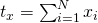And here is the procedure

1. We assumeis known,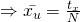is known
2. Use SRS and measureandin the sample.
3. Calculateandfor the sample
4.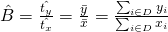5.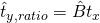6.We use ratio estimation because at times, our  ratio of interest might be average yield in bushels per acre, ratio of fish caught to the number of hours spend, per capita income, etc. And for most of these cases, the population size N is unknown, so its still necessary for us to estimate a population total. Since we cannot use the estimatorhere, we consider another measure of size, that is,. So we can estimate N by. Consequently,whereestimates the total sample size based on the auxiliary variable.

The benefits of using ratio estimation is clear.

• Smaller MSE if x and y are correlated, giving us an increase of precision
• We are able to adjust estimates to reflect known information, and evaluate them more in depth for a more representative result.
• We can adjust for nonresponse.

You might notice that taking a SRS will slight underestimate the true population mean of x‘s, that is,is smaller than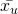. And should x and y be positively correlated,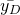may also underestimate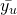Ratio estimation for the population mean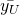is given byHere we correct the underestimation by expandingby a factor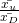After looking at the estimators, as usual, we questions its qualities.

Firstly, the ratio estimators are biased. This arises because the unbiasedis multiplied by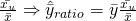. The good news is that our variance is reduced, essentially compensating for the presence of bias. This means that although, the value offor any individual sample is likely to be closer tothan the sample mean. Of course, the average deviation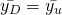, averaged over all possible samples D that could be obtained, is zero.

We introduce a population correlation coefficient of x and first.=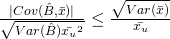==whereHere, notice that as sample size increased,decreases. Ignoring FPC, then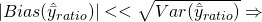MSE is dominated by the variance. So in large samples,Let, then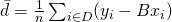is an unbiased estimator forWhen n is large (more than 30),Its is worth asking ourselves when this approximate MSE is small. Rewriting it, we have.
So approximate MSE is small when

• Sample size n is small
• sampling fractionis large
• Deviationsare small
• Correlation between x and y is close to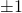•is large.

Estimated variance,where=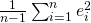andWhenis unknown, we can substitute it by, then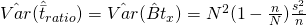Similarly, if the sample sizes are sufficiently large, approximate 95% CIs can be constructed using the standard errors asFor large samples, the effect of bias in the CIs can be ignored.

A distinct advantage of using ratio estimation is that the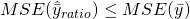iff. This implies that if the coefficient of variation are approximately equal, then it pays to use ratio estimation when the correlation between x and y is larger thanNext time, we will look at Regression Estimation.

Sampling & Survey #1 – Introduction
Sampling & Survey #2 – Simple Probability Samples
Sampling & Survey #3 – Simple Random Sampling
Sampling & Survey #4 – Qualities of estimator in SRS
Sampling & Survey #5 – Sampling weight, Confidence Interval and sample size in SRS
Sampling & Survey #6 – Systematic Sampling
Sampling & Survey #7 – Stratified Sampling
Sampling & Survey # 8 – Ratio Estimation
Sampling & Survey # 9 – Regression Estimation
Sampling & Survey #10 – Cluster Sampling
Sampling & Survey #11 – Two – Stage Cluster Sampling
Sampling & Survey #12 – Sampling with unequal probabilities (Part 1)
Sampling & Survey #13 – Sampling with unequal probabilities (Part 2)
Sampling & Survey #14 – Nonresponse

Not readable? Change text.